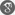##### Cite as:
K. R. Prasad, B. M. B. Krushna, N. Sreedhar, Eigenvalues for Iterative Systems of (n,p)-Type Fractional Order Boundary Value Problems, Int. J. Anal. Appl., 5 (2) (2014), 136-146.

#### Abstract

In this paper, we determine the eigenvalue intervals of λ1, λ2, ..., λn for which the iterative system of (n,p)-type fractional order two-point boundary value problem has a positive solution by an application of Guo-Krasnosel’skii fixed point theorem on a cone.

#### References

1. Z. Bai and H. Lu, Positive solutions for boundary value problems of nonlinear fractional differential equations, J. Math. Anal. Appl., 311(2005), 495-505.2. M. Benchohra, J. Henderson, S. K. Ntoyuas and A. Ouahab, Existence results for fractional order functional differential equations with infinite delay, J. Math. Anal. Appl., 338(2008), 1340-1350.3. J. M. Davis, J. Henderson, K. R. Prasad and W. Yin, Eigenvalue intervals for non-linear right focal problems, Appl. Anal., 74(2000), 215-231.4. L. H. Erbe and H. Wang, On the existence of positive solutions of ordinary differential equations, Proc. Amer. Math. Soc., 120(1994), 743-748.5. D. Guo and V. Lakshmikantham, Nonlinear Problems in Abstract Cones, Academic Press, Orlando, 1988.6. J. Henderson and S. K. Ntouyas, Positive solutions for systems of n th order three-point nonlocal boundary value problems, Electronic Journal of Qualitative Theory of Differential Equations, 18(2007), 1-12.7. J. Henderson and S. K. Ntouyas, Positive solutions for systems of nonlinear boundary value problems, Nonlinear Studies, 15(2008), 51-60.8. J. Henderson, S. K. Ntouyas and I. K. Purnaras, Positive solutions for systems of generalized three-point nonlinear boundary value problems, Comment. Math. Univ. Carolin., 49, 1(2008), 79-91.9. J. Henderson, S. K. Ntouyas and I. K. Purnaras, Positive solutions for systems of second order four-point nonlinear boundary value problems, Commu. Appl. Anal., 12(2008), No.1, 29-40.10. E. R. Kauffman and E. Mboumi, Positive solutions of a boundary value problem for a nonlinear fractional differential equation, Electronic Journal of Qualitative Theory of Differential Equations, 3(2008), 1-11.11. R. A. Khan, M. Rehman and J. Henderson, Existence and uniqueness of solutions for nonlinear fractional differential equations with integral boundary conditions, Fractional Differential Calculus, 1(2011), 29-43.12. A. A. Kilbas, H. M. Srivasthava and J. J. Trujillo, Theory and Applications of Fractional Differential Equations, North-Holland Mathematics Studies, vol. 204, Elsevier, Amsterdam, 2006.13. M. A. Krasnosel’skii, Positive Solutions of Operator Equations, Noordhoff, Groningen, 1964.14. I. Podulbny, Fractional Differential Equations, Academic Press, San Diego, 1999.15. K. R. Prasad and B. M. B. Krushna, Multiple positive solutions for a coupled system of Riemann-Liouville fractional order two-point boundary value problems, Nonlinear Studies, Vol. 20, No.4(2013), 501-511.16. X. Su and S. Zhang, Solutions to boundary value problems for nonlinear differential equations of fractional order, Electronic Journal of Differential Equations, 26(2009), 1-15.17. S. Zhang, Existence of solutions for a boundary value problem of fractional order, Acta Math. Sci., 26B(2006), 220-228.